CE 338 2021 Spring
Final Project: Design of SAR Block
Nic Theobald
nstheobald@fortlewis.edu

Simple 8-bit SAR block

### Introduction

This lab covers the simulation of the Succesive Approximation Register (SAR) block for a SAR ADC. This block successivly shifts the results of a comparator to the SAR's output. This allows you to approximate an analog voltage within 2^n divisions.

## Methods and Materials

 Item Quantity LTspice Electric VLSI Ideal ADC/DAC Library 1 1 1

The SAR block was built out of D flip flops which were built from 3 input Nand Gates. 50 nm technology was used for this lab. A VCC of 1v was used.

## Results

Task 1: Create 3 input Nand

The 2 input nand was first created using 50nm technology. The 2 input nand was turned into a symbol, which was used to create the 3 input nand.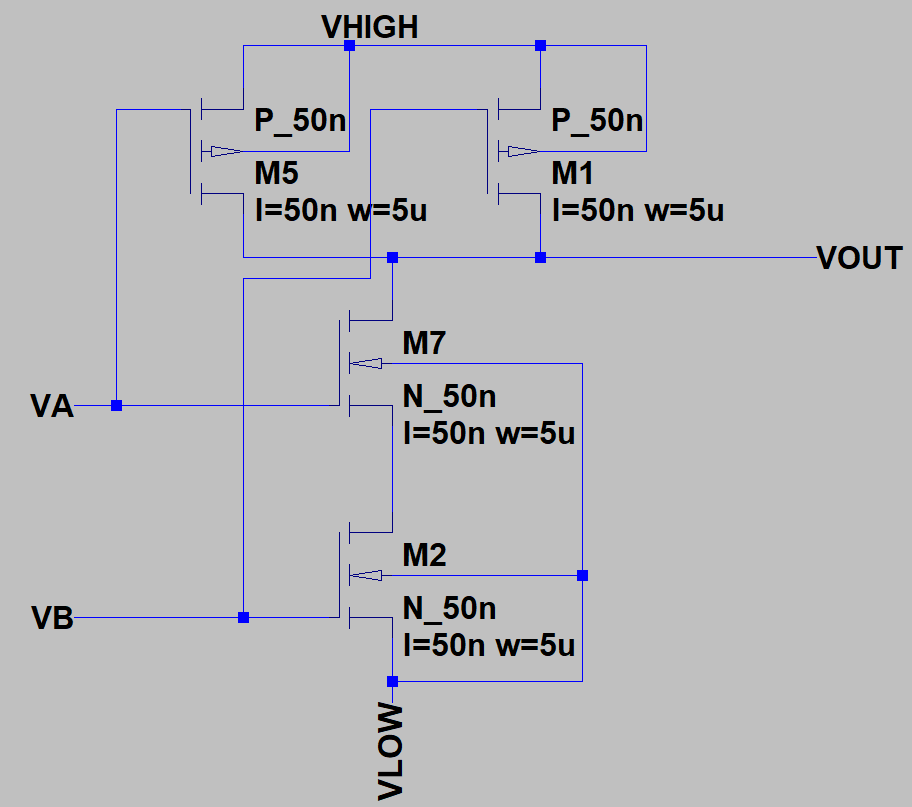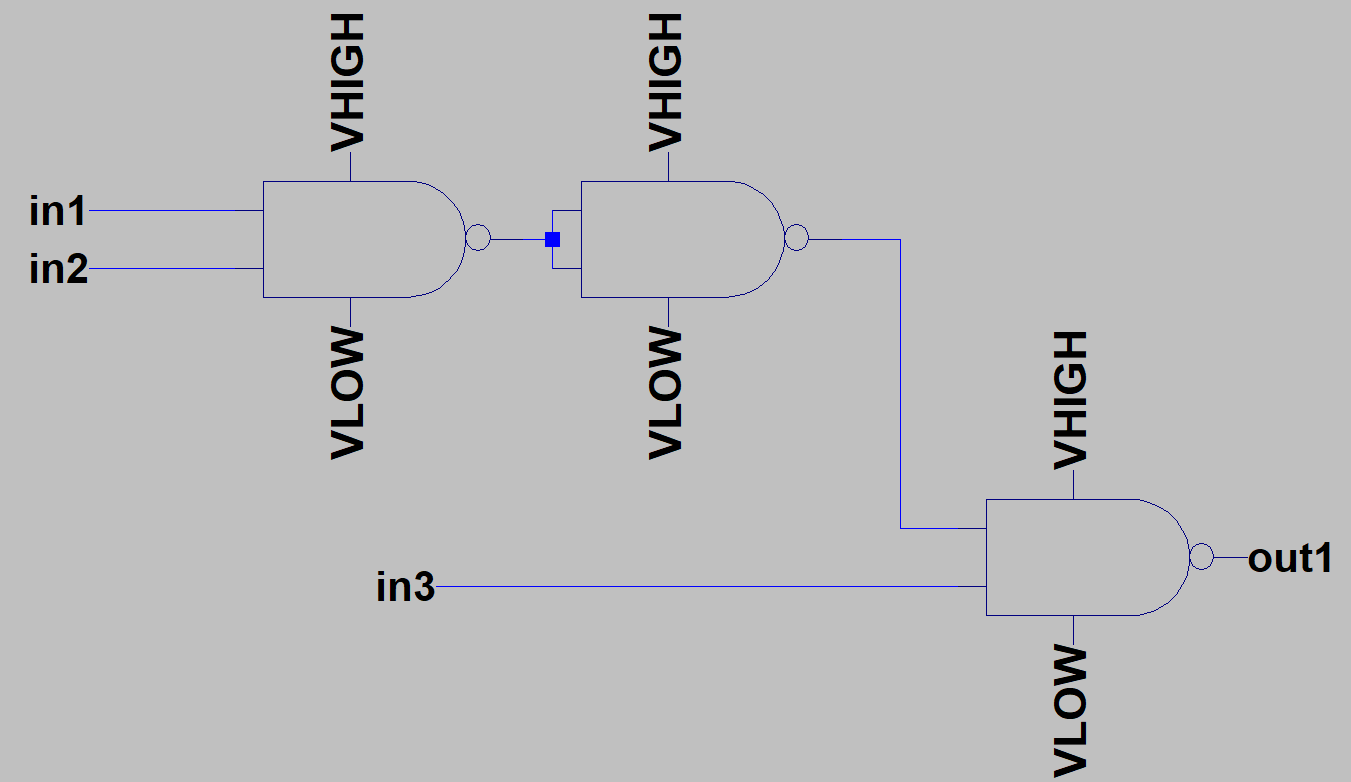Figure 1: Creation of 3 input nand.

The 3 input nand was then simulated.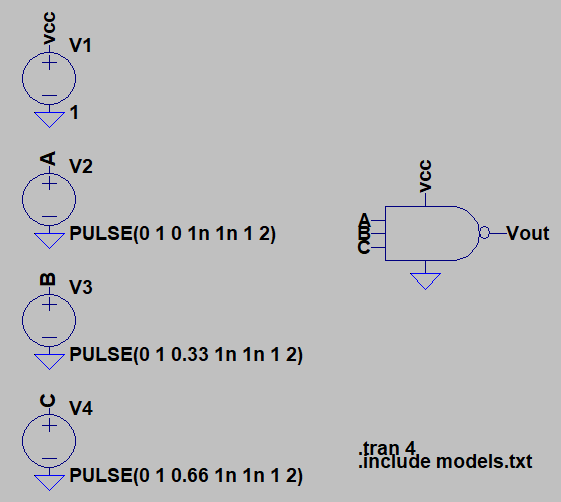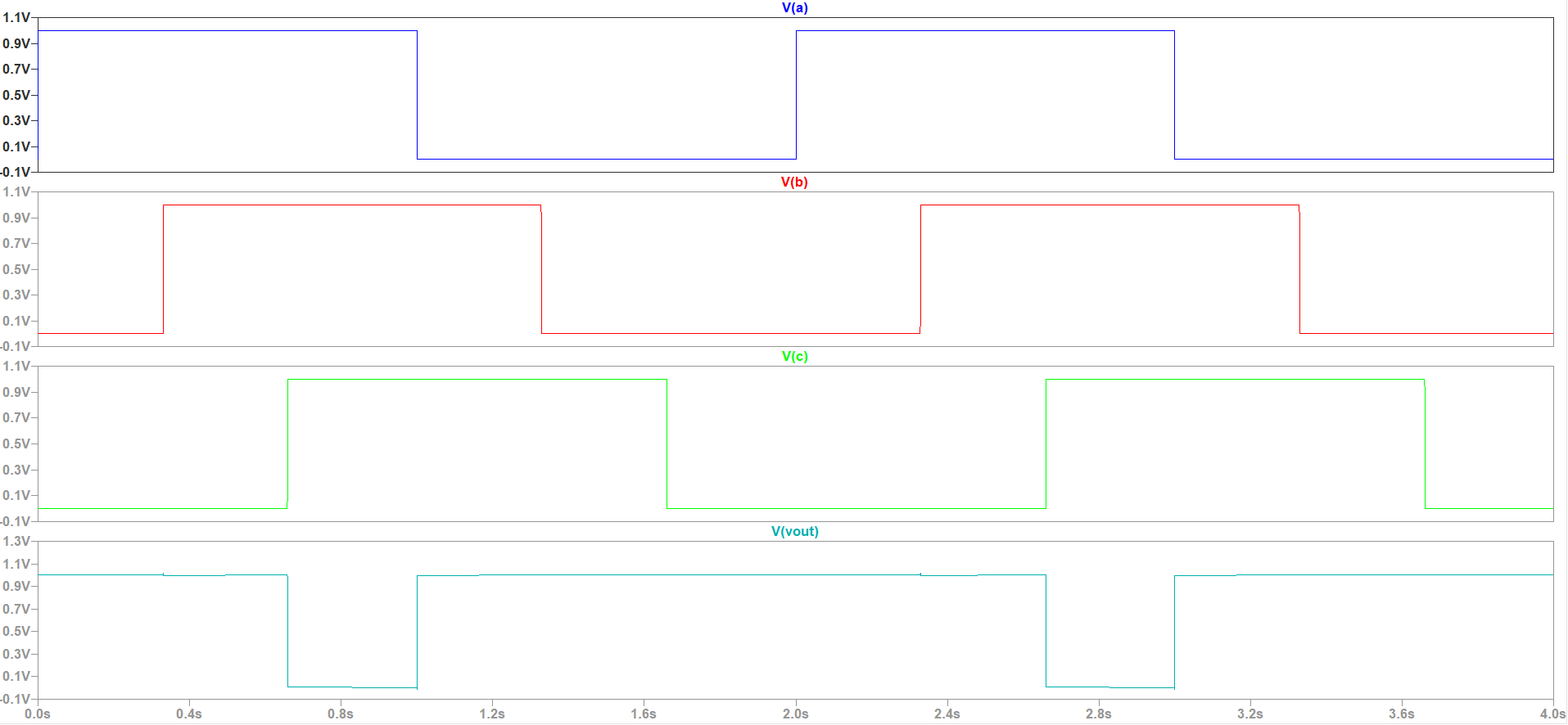Figure 2: Simulation of 3 input nand.

Task 2: Create DFF using 3 input nand

The D flip flop was created from 3 input nand.Figure 3: DFF from 3 input nand.

The DFF was packaged into a symbol and was simulated.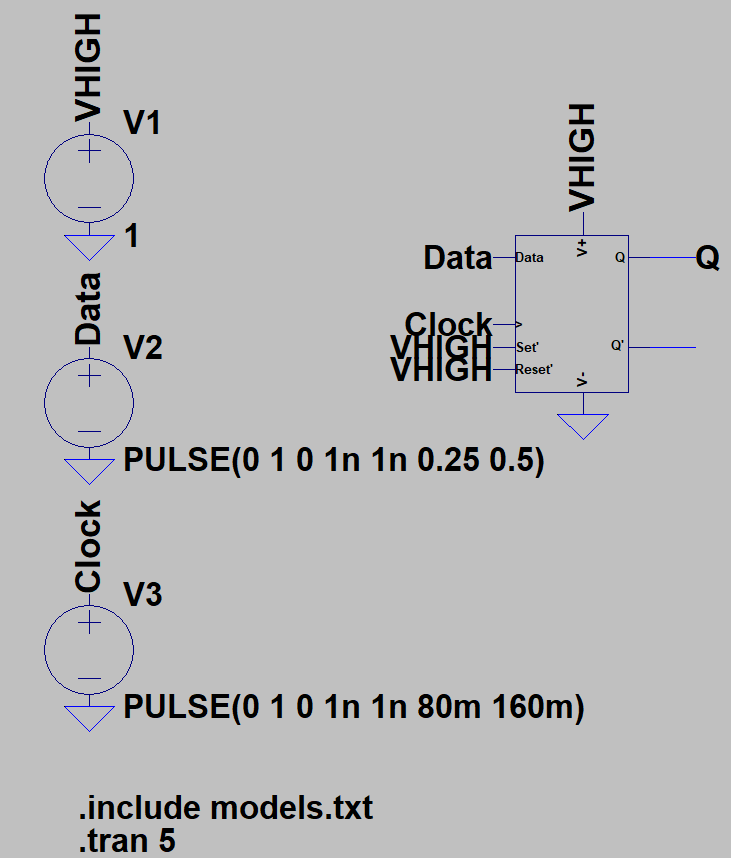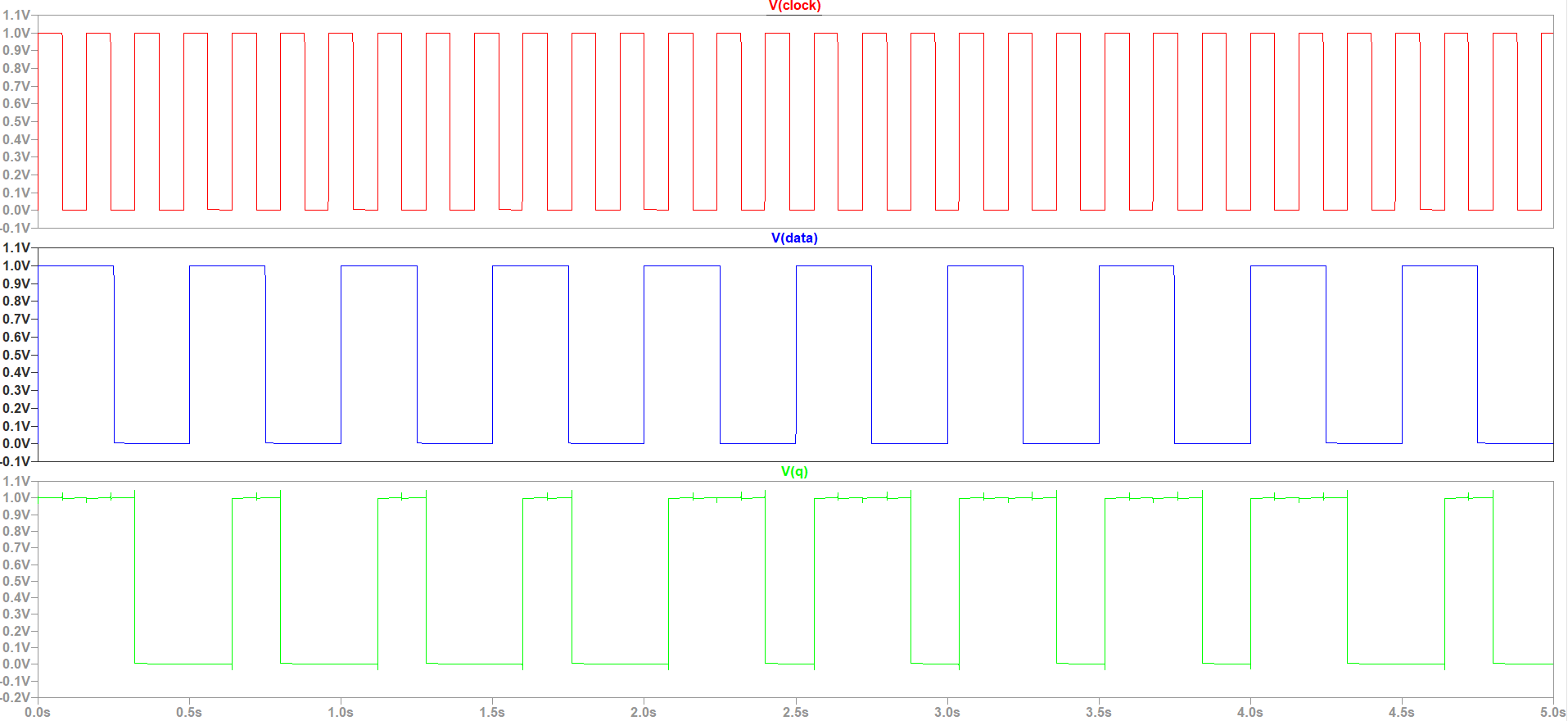Figure 4: Simulation of DFF.

## Task 3: Create SAR block using DFF

The SAR block was created using the DFF.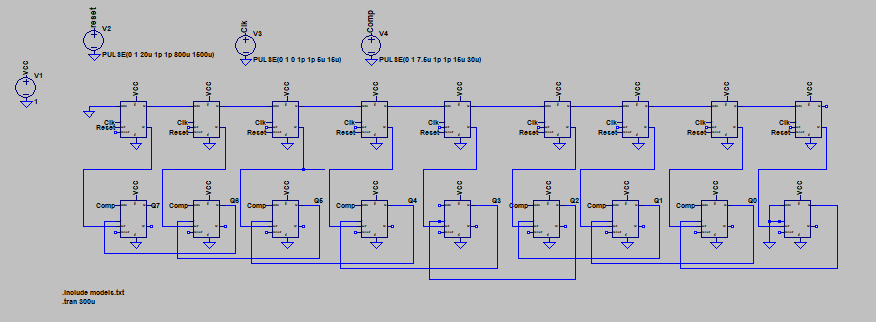Figure 5: Sar block from DFF.

The SAR block was packaged into a symbol and simulated.Figure 6: Simulation of SAR block.

## Discussion:

This lab detailed the design and simulation of an 8 bit SAR block.